### Home > ACC7 > Chapter 9 Unit 10 > Lesson CC3: 9.1.1 > Problem9-13

9-13.

Determine whether the graphs below are functions or not functions. Explain your reasoning.

A graph is only a function if there is no more than one $y$-value for any given $x$-value.

1.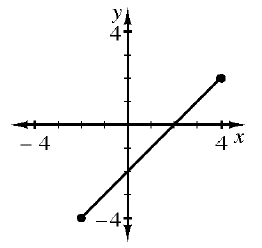Are there any points on this line segment that are above or below another point on the line segment? If there are, the graph is not a function.

This graph is a function.

1.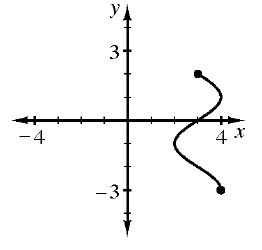See hint for part (a).

This graph is not a function. Some $x$-values are related to multiple $y$ values. For example, when $x$ is $3$, $y$ can be $2$ or $≈ 2$.

1.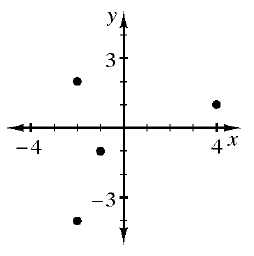See hint for part (a).

1.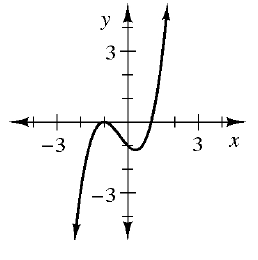See hint for part (a).

1.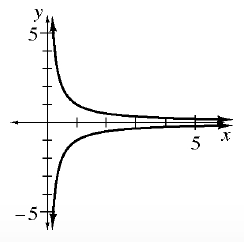See hint for part (a).

This graph is not a function.

1.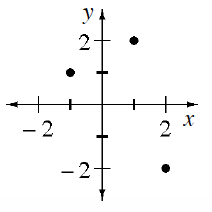See hint for part (a).